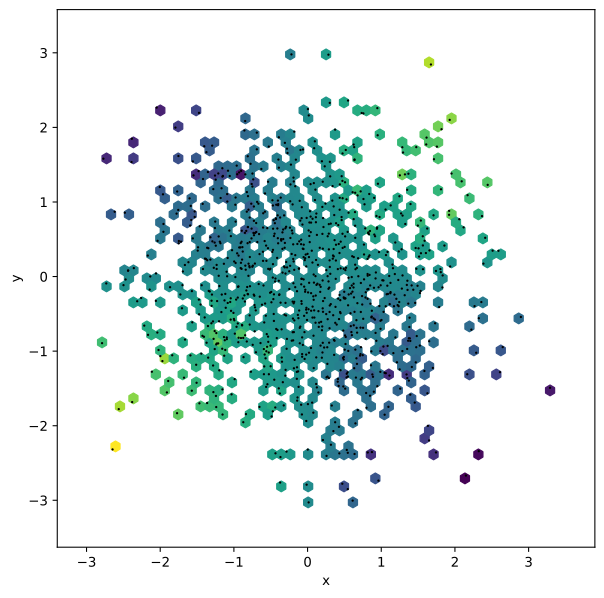# HexTiles#

## Title: HexTiles Element#

Dependencies Matplotlib

Backends Matplotlib, Bokeh

```import numpy as np
import holoviews as hv
from holoviews import opts

hv.extension('matplotlib')
hv.output(fig='svg', size=200)
````HexTiles` provide a representation of the data as a bivariate histogram useful for visualizing the structure in larger datasets. The `HexTiles` are computed by tesselating the x-, y-ranges of the data, storing the number of points falling in each hexagonal bin. By default `HexTiles` simply counts the data in each bin but it also supports weighted aggregations. Currently only linearly spaced bins are supported when using the bokeh backend.

As a simple example we will generate 100,000 normally distributed points and plot them using `HexTiles`:

```np.random.seed(44)
hex_tiles = hv.HexTiles(np.random.randn(100000, 2))
hex_tiles.opts(colorbar=True)
```Note that the hover shows a `Count` by default, representing the number of points falling within each bin.

It is also possible to provide additional value dimensions and define an `aggregator` to aggregate by value. If multiple value dimensions are defined the dimension to be colormapped may be defined using the `size`. Note however that multiple value dimensions are allowed and will be displayed in the hover tooltip.

```xs, ys = np.random.randn(2, 1000)
hex_with_values = hv.HexTiles((xs, ys, xs*(ys/2.), (xs**2)*ys), vdims=['Values', 'Hover Values'])
points = hv.Points(hex_with_values)

(hex_with_values * points).opts(
opts.HexTiles(aggregator=np.sum),
opts.Points(s=3, color='black'))
```By default `HexTiles` do not plot bins that do not contain any data but using the `min_count` option it is possible to plot bins with zero values as well or alternatively set a higher cutoff. Using this options can result in a more visually appealing plot particularly when overlaid with other elements.

Here we will plot the same distributions as above and overlay a `Bivariate` plot of the same data:

```x, y = np.hstack([np.random.randn(2, 10000), np.random.randn(2, 10000)*0.8+2])
hex_two_distributions = hv.HexTiles((x, y))
bivariate = hv.Bivariate((x, y))

(hex_two_distributions * bivariate).opts(
opts.Bivariate(linewidth=3, show_legend=False),
opts.HexTiles(min_count=0))
```